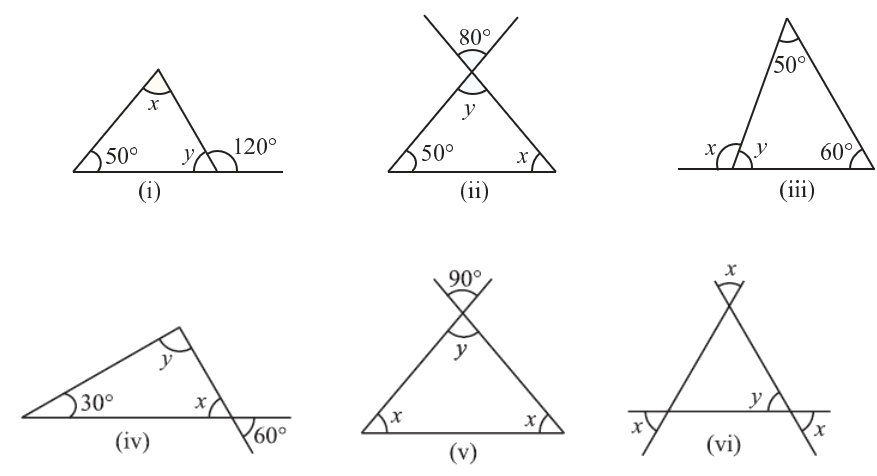# Ex.6.3 Q2 The-Triangle-and-its-Properties Solution - NCERT Maths Class 7

Go back to  'Ex.6.3'

## Question

Find the values of the unknowns $$x$$ and $$y$$ in the following diagrams:Video Solution
Triangle & Its Properties
Ex 6.3 | Question 2

## Text Solution

What is known?

Measure of one interior angle and exterior angle.

What is unknown?

Value of $$x$$ and $$y$$ in the given diagrams

Reasoning:

Let us assume the unknown interior angle of the given triangle as $$“x”$$ and $$“y”.$$ Now, let us model this problem visually. We must know the two properties.

1. Keeping in mind that sum of the interior opposite angles of a triangle is always equal to the exterior angle, we can find out the value of unknown interior value.
2. Knowing the angle sum property i.e. sum of the interior angles of a triangle is always equal to$$180^\circ$$, we can then find out another unknown interior angle.

Steps:

(i) We know that, Sum of interior opposite angles of a triangle $$=$$ Exterior angle

\begin{align} 50^\circ+x&=120^\circ\\ x&=120^\circ-50^\circ\\ x&=70^\circ\end{align}

By angle sum property,

Sum of interior angles $$=$$ $$180^\circ$$

\begin{align}50^\circ+70^\circ+y&=180^\circ\\ 120^\circ+y&=180^\circ\\ y&=180^\circ-120^\circ\\ y&=60^\circ\end{align}

(ii)

What is known?

Measure of one interior angle and exterior angle.

What is unknown?

Value of $$“x”$$ & $$“y”.$$

Reasoning:

This question is simple based on two concepts. First vertically opposite angles are equal by using this we can find out the angle opposite to $$80^\circ$$ i.e. one of the interior angles. Now, one more interior angle is unknown here so, we use the angle sum property i.e. sum of three interior angles of a triangle is $$180^\circ$$ and find out the value of another unknown angle.

Steps:

Solve for $$x$$ and $$y$$

\begin{align} y=80^\circ \end{align} (vertically opposite angles)

By angle sum property

Sum of interior angles $$=$$ $$180^\circ$$

\begin{align}50^\circ+x+y&=180^\circ\\ 50^\circ+x+80^\circ&=180^\circ\\ 130^\circ+x&=180^\circ\\ x&=180^\circ-130^\circ \\ x&=50^\circ\end{align}

(iii)

What is known?

Measure of two interior angles.

What is unknown?

Value of $$“x”$$& $$“y”.$$

Reasoning:

Visually identify the unknown interior angle and then follow two steps. First, by using angle sum property we can find out the value of unknown interior angle and then for angle $$x$$ we know that sum of interior opposite angles of a triangle is equal to exterior angle.

Steps:

Solve for $$x$$ and $$y$$

We know that, Sum of interior opposite angles of a triangle $$=$$ Exterior angle

\begin{align}50^\circ+60^\circ&=x\\110^\circ&=x\\x&=110^\circ\end{align}

By angle sum property,

Sum of interior angles $$=$$$$180^\circ$$

\begin{align} 50^\circ+60^\circ+y&=180^\circ\\110^\circ+y&=180^\circ\\y&=180^\circ-110^\circ\\y&=70^\circ\end{align}

(iv)

What is known?

Measure of one interior angle and exterior angle.

What is unknown?

Value of $$“x”$$ & $$“y”.$$

Reasoning:

This question is simple based on two concepts. First vertically opposite angles are equal by using this we can find out the angle opposite to $$60^\circ$$ i.e. one of the interior angles. Now, one more interior angle is unknown here so, we use the angle sum property i.e. sum of three interior angles of a triangle is $$180^\circ$$ and find out the value of another unknown angle.

Steps:

Solve for $$x$$and $$y$$

$$x\! =\!60^0$$ (vertically opposite angles are equal)

We know that,

Sum of interior angles of a triangle $$=$$$$180^\circ$$

\begin{align} 30^\circ+x+y&=180^\circ\\30^\circ+60^\circ+y&=180^\circ\\90^\circ+y&=180^\circ\\y &=180^\circ-90^\circ\\y&=90^\circ\end{align}

(v)

What is known?

Measure of Exterior angle.

What is unknown?

Value of $$“x”$$ & $$“y”.$$

Reasoning:

This question is simply based on two concepts. First, vertically opposite angles are equal by using this we can find out the value of one interior angle and then by applying angle sum property we can find out another interior angle. Here the measure of two interior angles is equal.

Steps:

Solve for $$x$$ and $$y$$

$$y=90^0$$ (vertically opposite angles are equal)

We know that,

Sum of interior angles of a triangle $$=$$$$180^\circ$$

\begin{align} y+ x+x&=180^\circ\\90^\circ + 2x&=180^\circ\\2x&=180^\circ-90^\circ\\ x&=\frac{90{}^\circ }{2} \\ x&=45{}^\circ \\ \end{align}

(vi)

What is unknown?

Two angles i.e. $$“x”$$& $$“y”.$$

Reasoning:

This question is simply based on two concepts. First, vertically opposite angles are equal by using this we can find out that $$x$$ and $$y$$ are equal and then by applying angle sum property we can find all angles.

Steps:

Solve for $$x$$and $$y$$

$$x = y$$ (vertically opposite angles are equal)

We know that,

Sum of interior angles of a triangle $$=180^\circ$$

\begin{align}x+x+y&= 180^\circ\\ 2x+y &=180^\circ\\2x+x &=180^\circ\qquad (x = y)\\3x &=180^\circ\\ x &=\frac{180{}^\circ }{3} \\ x &=60{}^\circ \\ \end{align}

Since $$x = y$$

Therefore,

$$y=60^\circ$$

Reasoning Shortcut / Tip: Whenever you encounter problems of this kind, its best to recall the angle sum property of a triangle.

Learn from the best math teachers and top your exams

• Live one on one classroom and doubt clearing
• Practice worksheets in and after class for conceptual clarity
• Personalized curriculum to keep up with school